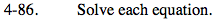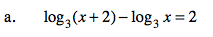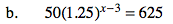### Home > PC > Chapter 4 > Lesson 4.2.2 > Problem4-86

4-86.Rewrite using one log:

Undo.

Solve for x.

$\text{log}_3\frac{x+2}{x}=2$

$\small 3^{\text{log}_{3}\frac{x+2}{x}}=3^2$

$\frac{x+2}{x}=9$

$x+2=9x$

$2 = 8x$

$x = \frac{1}{4}$1. Divide both sides by 50.
2. Take the log of both sides bring the exponent down.
3. Solve for x.